8011

Mathematics Trigonometry Level: Misc Level

Solve cos ø + 2cos2 ø = 0 given that 0o < 0 <360o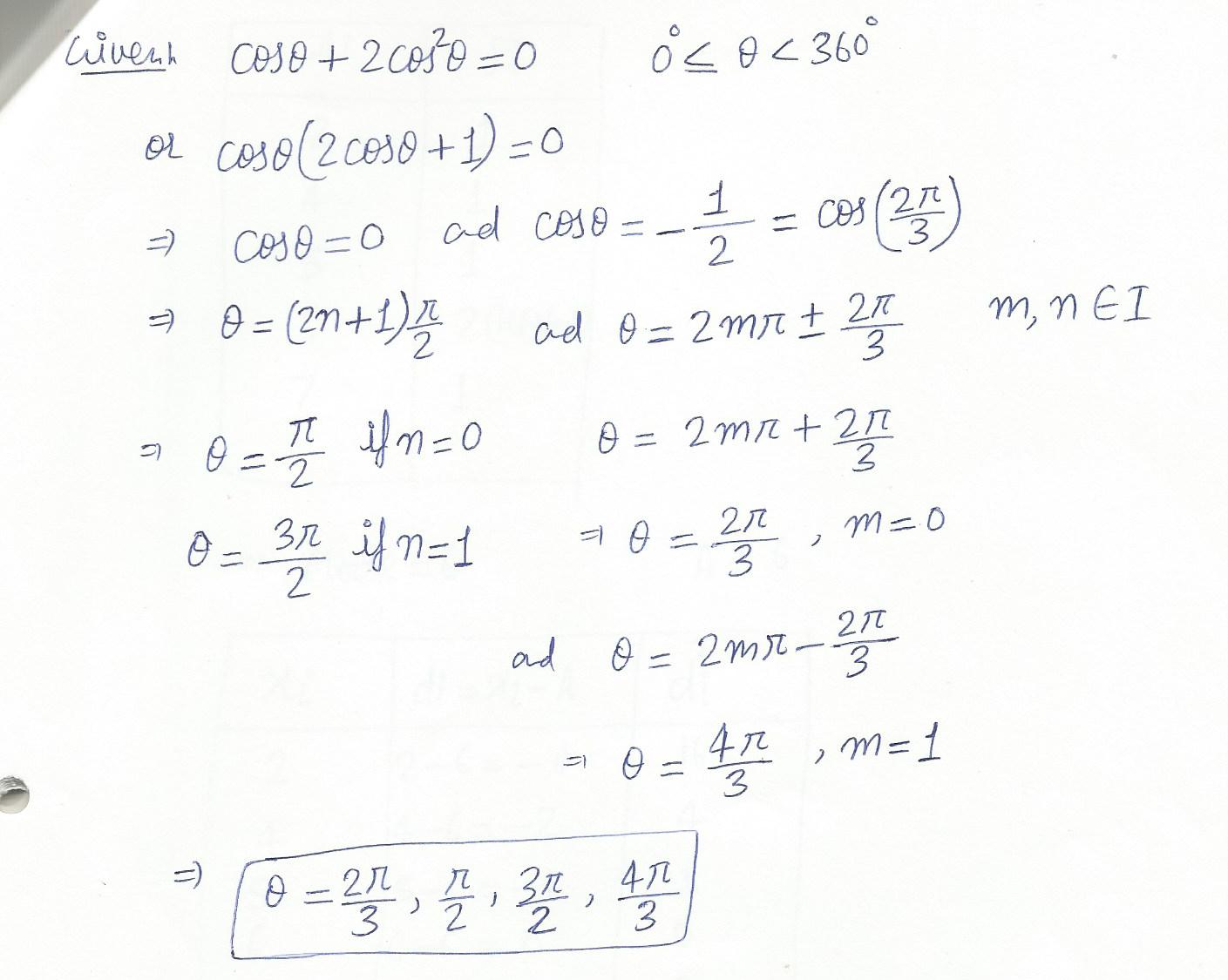6075

Mathematics Trigonometry Level: Misc Level

The corners of an equilateral triangle lie on a circle of radius 2.84 m. Calculate the length of side of the triangle.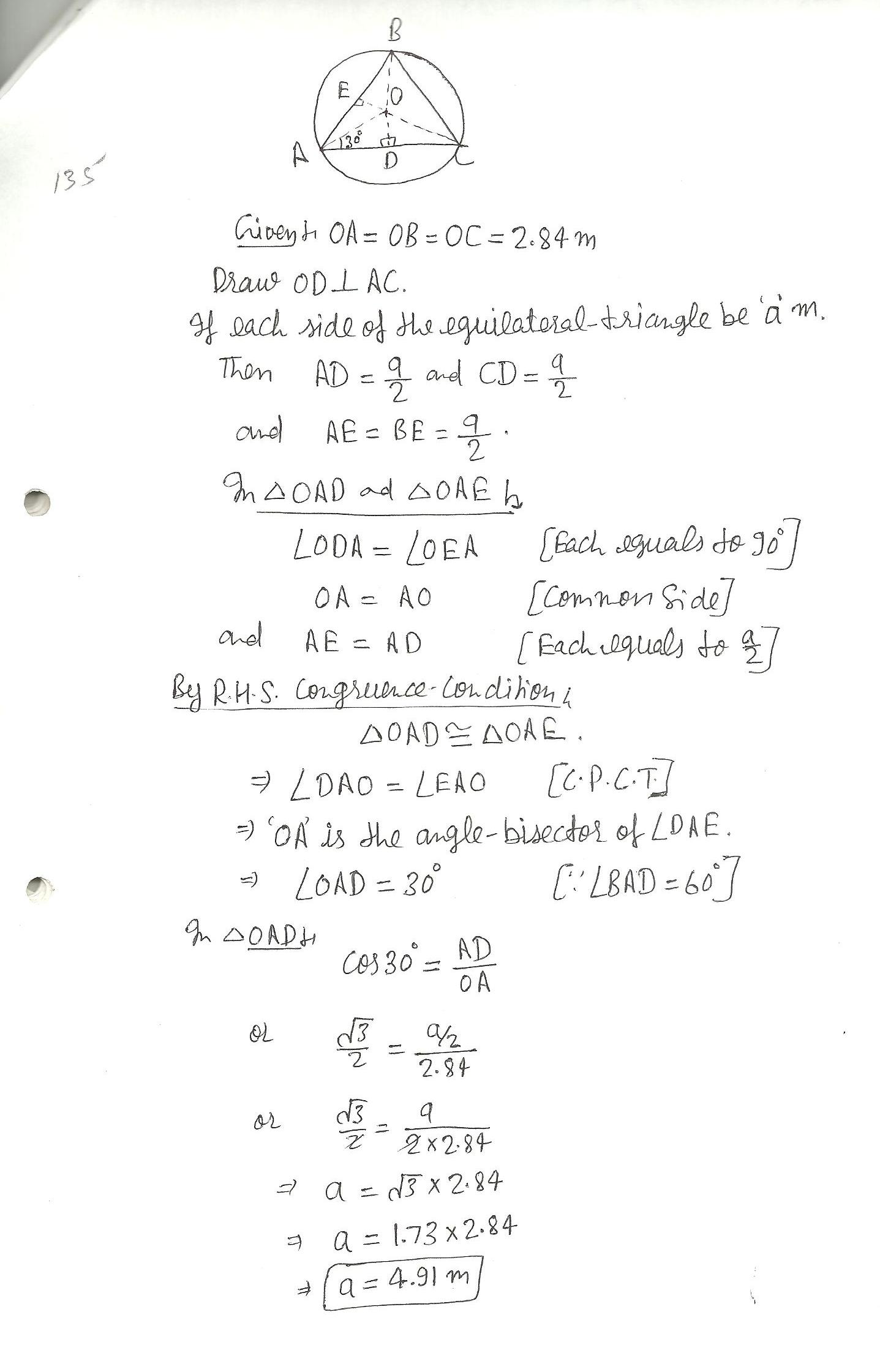6073

Mathematics Trigonometry Level: Misc Level

A  lighthouse that rises h1=50.6 ft above the surface of the water sits on a rocky cliff that extends d =20.1 ft from its base, as shown in the figure below. A sailor on the deck of a ship sights the top of the lighthouse at an angle of 0= 31.5% above the horizontal.If the sailor,s eye level is h2=14.0 ft above the water, how far is the ship from the rocks?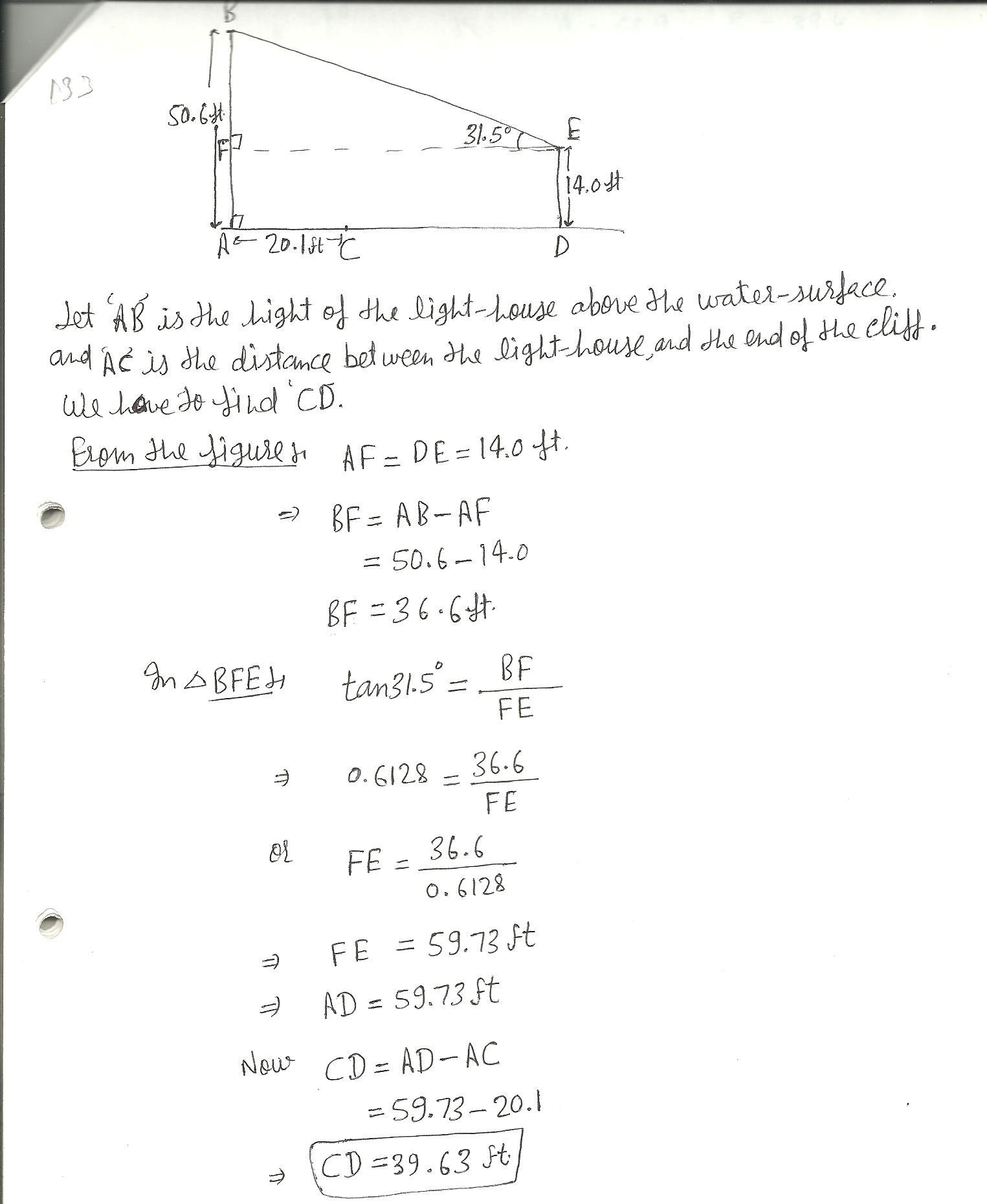5093

Mathematics Trigonometry Level: Misc Level

A triangle has angles of 1.02 radians and 0.85 radians. What is the third angle?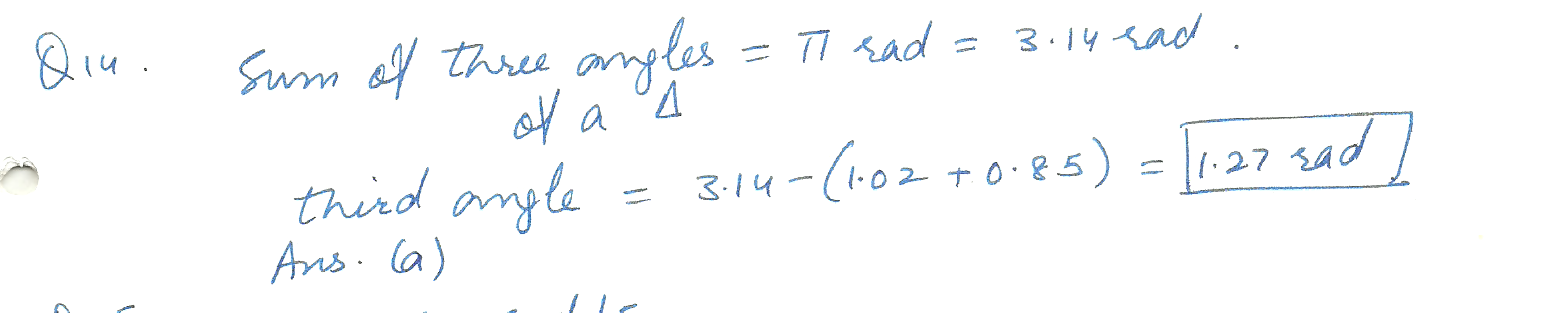4080

Mathematics Trigonometry Level: Misc Level

6. prove cos 2ly +1/ coty =SIN 2y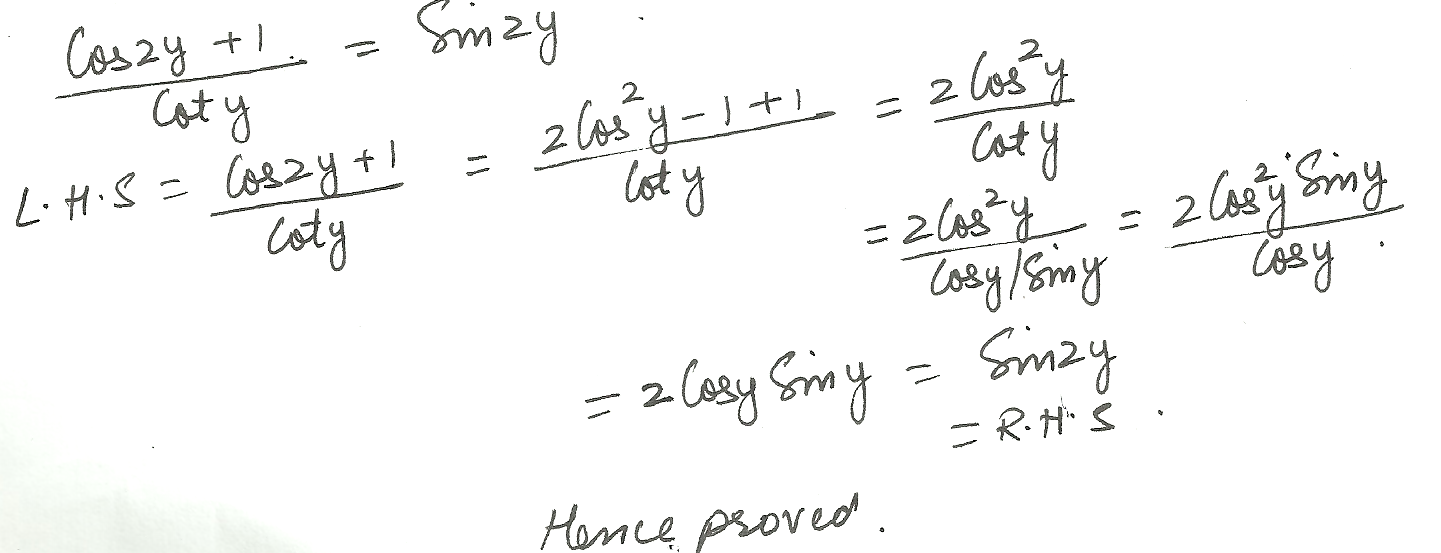4079

Mathematics Trigonometry Level: Misc Level

5. if SIN A =.1 and COS A= - squate root of . 99, then A) SIN (A+pie/6) B) COS (pie/4-A)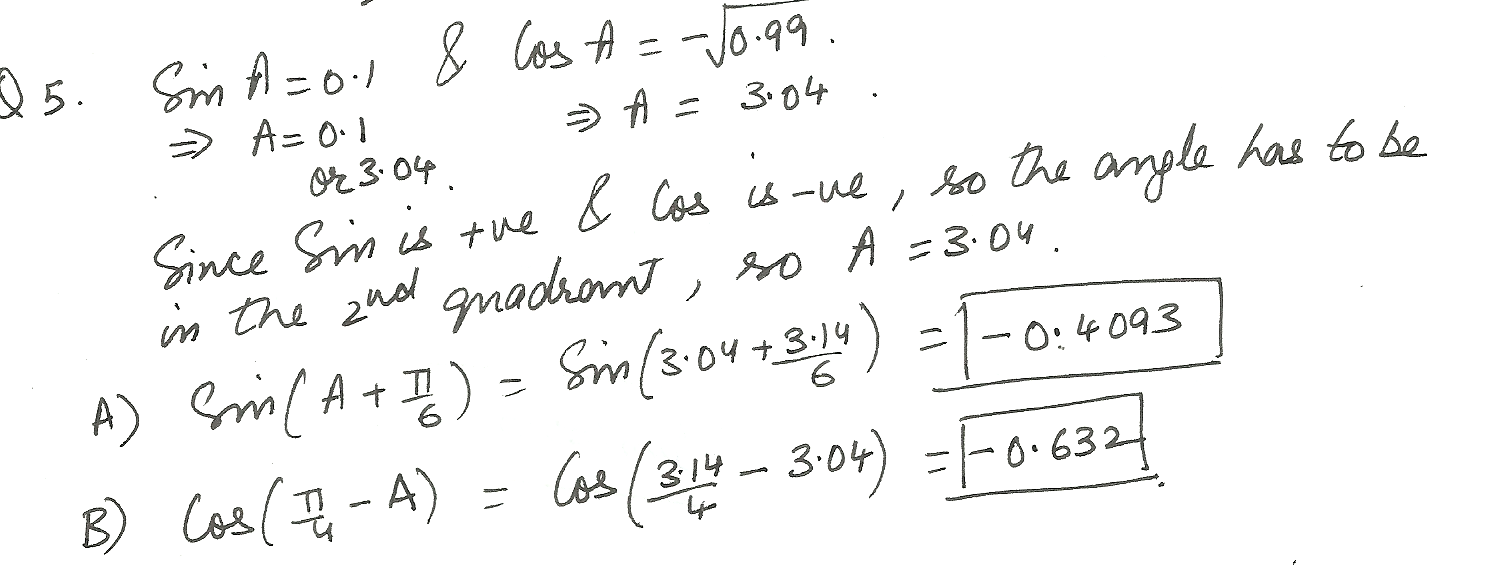4078

Mathematics Trigonometry Level: Misc Level

4. Given that Cos 0= - 7, pie <0 <3/ 2 pie. Find A) Sin 0/2 = B) Cos 0/2 =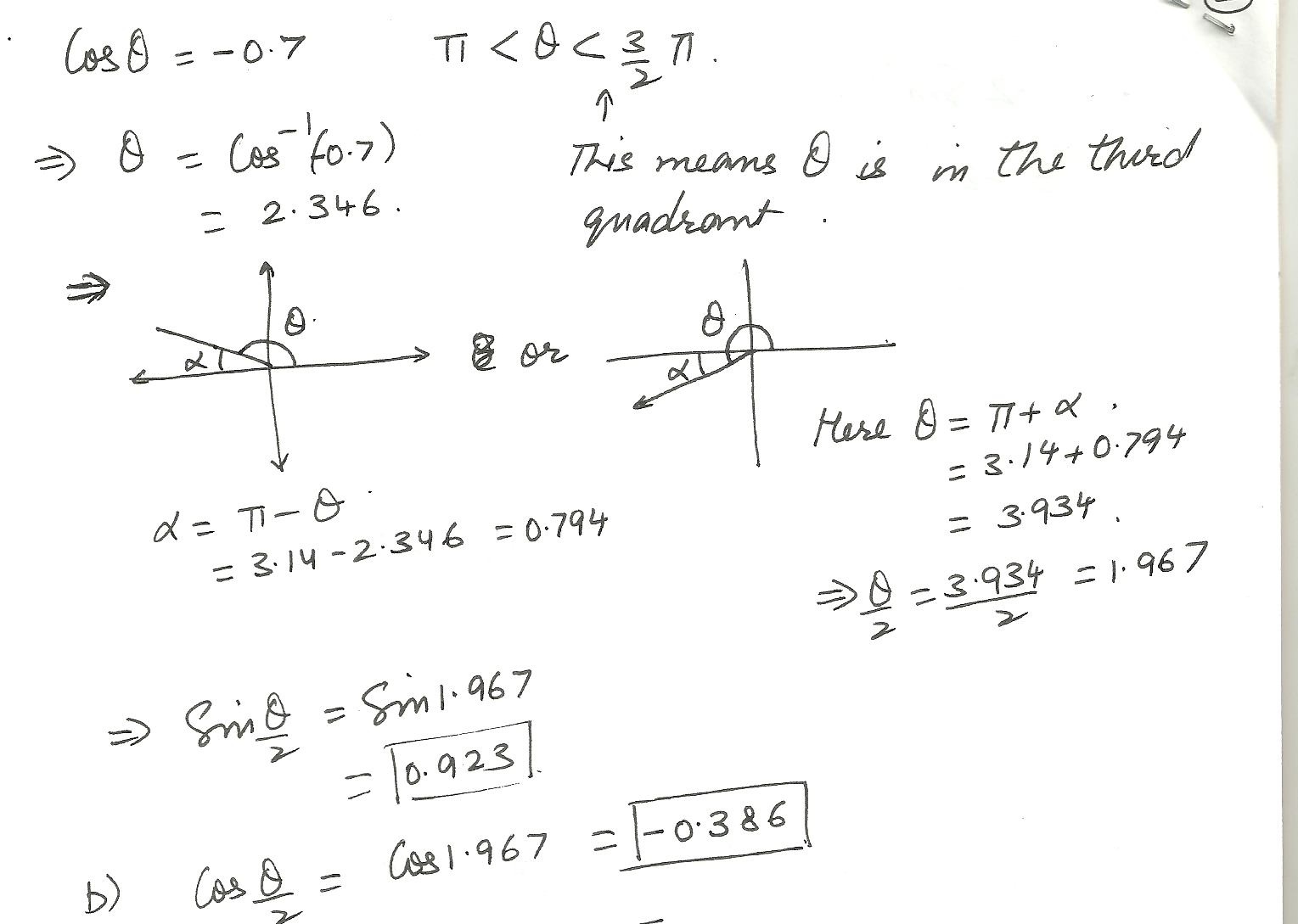4075

Mathematics Trigonometry Level: Misc Level

Questions: 1.Evaluate exactly

1-2 sin^2( 22.5^0)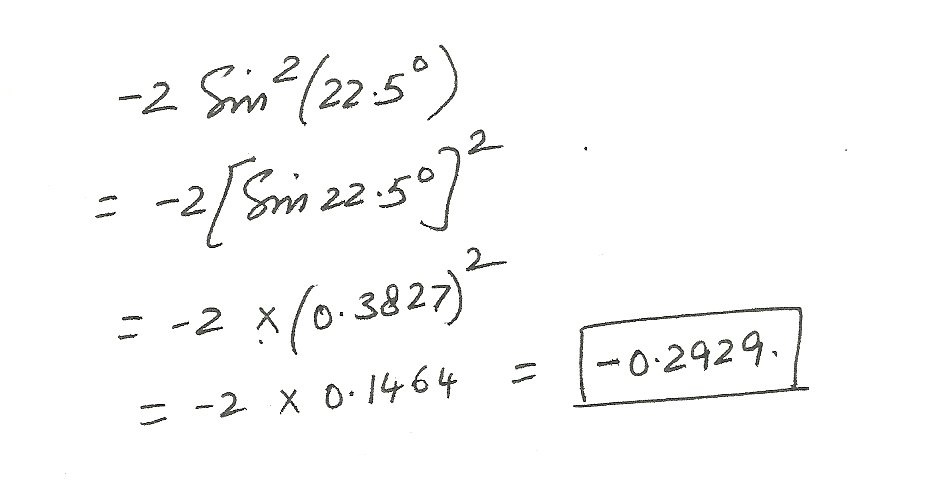3456

Mathematics Trigonometry Level: High School

Find all possible solution for the equation cos3x= (1/2)369

Mathematics Trigonometry Level: High School

The silhouette of a Christmas tree is an isosceles triangle . The angle at the top of the tree is 30 degrees , and the base measures 2.00 meters across . How tall is the tree ?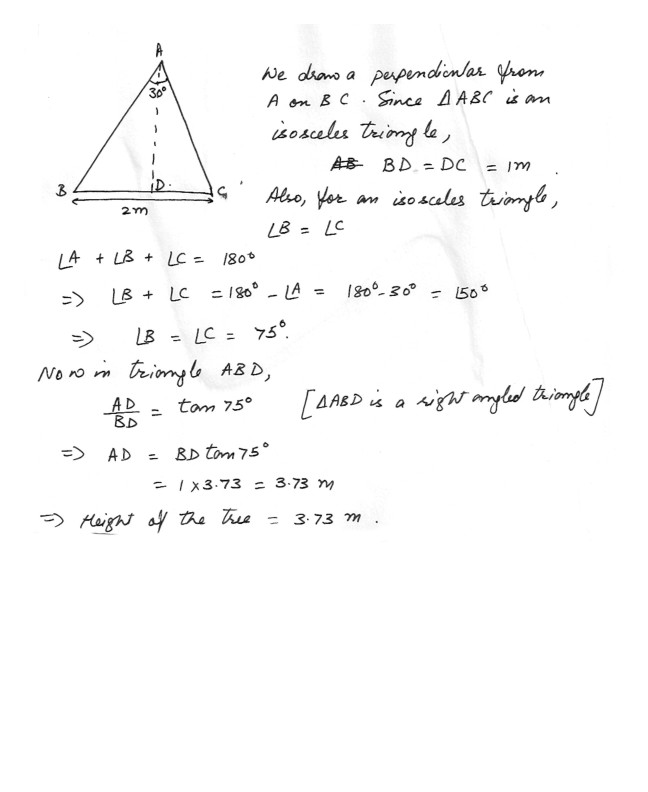368

Mathematics Trigonometry Level: High School

You are driving into St . Louis , Missouri , and in the distance you see the famous Gateway-to-the-west arch . This monument rises to a height of 192 meters . You estimate your line of sight with the top of the arch to be 2.0 degrees above the horizontal . Approximately how far (in km) are you from the base of the arch ?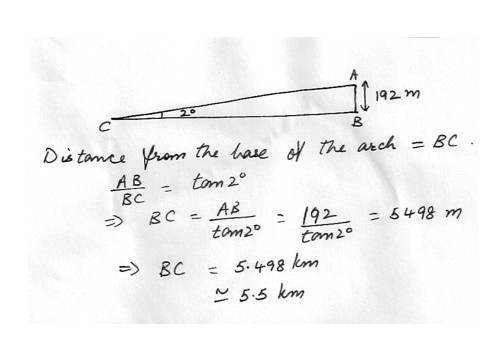Displaying 1-11 of 11 results.